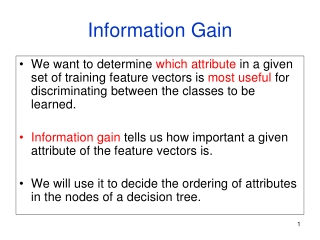DownloadDownload PresentationInformation Gain

# Information Gain

Télécharger la présentation## Information Gain

- - - - - - - - - - - - - - - - - - - - - - - - - - - E N D - - - - - - - - - - - - - - - - - - - - - - - - - - -
##### Presentation Transcript

1. Information Gain • We want to determine which attribute in a given set of training feature vectors is most useful for discriminating between the classes to be learned. • Information gain tells us how important a given attribute of the feature vectors is. • We will use it to decide the ordering of attributes in the nodes of a decision tree.

2. Calculating Information Gain Information Gain = entropy(parent) – [average entropy(children)] child entropy Entire population (30 instances) 17 instances child entropy parent entropy 13 instances (Weighted) Average Entropy of Children = Information Gain= 0.996 - 0.615 = 0.38 for this split

3. Entropy-Based Automatic Decision Tree Construction Node 1 What feature should be used? Training Set S x1=(f11,f12,…f1m) x2=(f21,f22, f2m) . . xn=(fn1,f22, f2m) What values? Quinlan suggested information gain in his ID3 system and later the gain ratio, both based on entropy.

4. Using Information Gain to Construct a Decision Tree 1 Choose the attribute A with highest information gain for the full training set at the root of the tree. Full Training Set S Attribute A 2 v2 v1 vk Construct child nodes for each value of A. Each has an associated subset of vectors in which A has a particular value. Set S  S={sS | value(A)=v1} 3 repeat recursively till when?

5. Simple Example Training Set: 3 features and 2 classes X Y Z C • 1 1 I 11 0 I 0 0 1 II 1 0 0 II How would you distinguish class I from class II?

6. X Y Z C • 1 1 I 11 0 I 0 0 1 II 1 0 0 II Split on attribute X If X is the best attribute, this node would be further split. I I II X=1 Echild1= -(1/3)log2(1/3)-(2/3)log2(2/3) = .5284 + .39 = .9184 I I II II II X=0 Echild2= 0 Eparent= 1 GAIN = 1 – ( 3/4)(.9184) – (1/4)(0) = .3112

7. X Y Z C • 1 1 I 11 0 I 0 0 1 II 1 0 0 II Split on attribute Y I I Y=1 Echild1= 0 I I II II II II X=0 Echild2= 0 Eparent= 1 GAIN = 1 –(1/2) 0 – (1/2)0 = 1; BEST ONE

8. X Y Z C • 1 1 I 11 0 I 0 0 1 II 1 0 0 II Split on attribute Z I II Z=1 Echild1= 1 I I II II I II Z=0 Echild2= 1 Eparent= 1 GAIN = 1 – ( 1/2)(1) – (1/2)(1) = 0 ie. NO GAIN; WORST